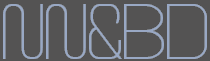# 1. 深度前馈网络

### 1.1 什么是深度前馈网络### 1.2 深度学习# 2. 梯度下降的再次学习

$w_{n+1}=w_{n}-\eta \frac{\partial E(w)}{\partial w}$

$E(w)'=0$

$E(f(w))'=E'(f(w))f'(w)$

$\frac{\partial E}{\partial w}=\frac{\partial E}{\partial y} \cdot \frac{\partial y}{\partial w}$

$\frac{\partial E}{\partial w}=\frac{\partial (t-O)^2}{\partial w}$

$\frac{\partial E}{\partial w_{j,k}}=\frac{\partial E}{\partial O_{k}} \cdot \frac{\partial O_{k}}{\partial w}$

$\frac{\partial E}{\partial w_{j,k}}=-2(t_{k}-O_{k})\frac{\partial O_{k}}{\partial w_{j,k}}$$O_{k}=Sigmoid({\sum_{k=1,j=1}^{4}} w_{j,k} \cdot x_{j})$

$\frac{\partial E}{\partial w_{j,k}}=-2(t_{k}-O_{k})\frac{\partial}{\partial w_{j,k}} Sigmoid(\sum w_{j,k} \cdot x_{j})$

$Sigmoid(x)=\frac{1}{1+e^{-x}}$

$Sigmoid$$x$的微分：

$\frac{\partial }{\partial x}Sigmoid(x)=Sigmoid(x)(1-Sigmoid(x))$$\frac{\partial E}{\partial w_{j,k}}=-(t_{k}-O_{k}) \cdot O_{k}\cdot (1-O_{k}) \cdot x_{j}$

$w_{n+1}=w_{n}-\eta \frac{\partial E}{\partial w_{j,k}}$

self.wih += self.lr * numpy.dot((output_errors * final_outputs * (1.0 - final_outputs)),
numpy.transpose(inputs))


# 3. 训练一个模型

## 3.1 监督学习简介1. 首先对模型参数进行初始化。通常采用对参数随机赋值的方法，但对于比较简单的模型，也可以将各参数的初值均设为0。
2. 读取训练数据（包括每个数据样本及其期望输出）。通常人们会在这些数据送入模型之前，随机打乱样本的次序。
3. 在训练数据上执行推断模型。这样，在当前模型参数配置下，每个训练样本都会得到一个输出值。
4. 计算残差。残差是一个能够刻画模型在最后一步得到的输出与来自训练集的期望输出之间差距的概括性指标。
5. 调整模型参数。在给定的损失函数条件下，学习的目的在于通过大量训练步骤改善各参数的值，从而将损失最小化。最常见的策略是使用梯度下降算法，也就是以上第二部分内容“再次学习梯度下降算法”所使用的方式。
6. 上述闭环会依据所需的学习速率、所给定的模型及其输入数据，通过大量循环不断重复上述过程。

## 3.2 简单说说无监督学习

1、《Python神经网络编程》
2、《深度学习》
3、《计算智能导论》
4、《面向机器智能的Tensorflow实践》
5、《机器学习导论》
6、https://www.zhihu.com/question/23194489

posted @ 2018-07-17 11:10  帅虫哥  阅读(3268)  评论(0编辑  收藏  举报